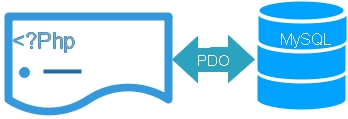# PHP PDO Fetch row from TableWE often need to collect one record from the table to display or to use in our script. To select one record we will use Pdo -> fetch to get next row from a result set. Here are some examples on how to use fetch on different conditions.
We kept our Pdo database connection details in config.php file and called it here.
``````
<?Php
error_reporting(E_ERROR | E_PARSE | E_CORE_ERROR);
require "config.php"; // Database connection details.
//////// End of Database connection /////////
\$id='3'; // Collecting one record with id=3
\$count=\$dbo->prepare("select * from pdo_admin where id=:id");
\$count->bindParam(":id",\$id,PDO::PARAM_INT,1);

if(\$count->execute()){
echo " Success <br>";
\$row = \$count->fetch(PDO::FETCH_OBJ);
print_r(\$row);
echo "<hr>";

}else{
//\$row=\$count->fetchAll();
print_r(\$dbo->errorInfo());
}
?>
``````
The output of above code is here
``````
( [id] => 3 [userid] => admin3 [password] => test3 [name] => Admin [status] => T )

pw =test3
``````
We have used print_r function to display the array
WE have used FETCH_OBJ parameter get property name corresponding to column name. Same way we will try other parameters In your downloaded script ( file name : pdo-fetch.php ) the area to change the part of the code is marked. You can replace them with these lines to check the output

## FETCH_NUM

We have to use column number starting from 0 in place of column name , omitted other lines and listed here the changes in above code.
``````\$row = \$count->fetch(PDO::FETCH_NUM);
print_r(\$row);
echo "<hr>";
echo "<br>userid = \$row";
``````
The output of above code will be
``````Array (  => 3  => admin3  => test3  => Admin  => T )
``````

## FETCH_ASSOC

Returned array will have column name ( field name ) as index. Here is the code
``````
\$row = \$count->fetch(PDO::FETCH_ASSOC);
print_r(\$row);
echo "<hr>";
echo "<br>userid = \$row[userid]";
``````
The output of the above is here
``````Array ( [id] => 3 [userid] => admin3 [password] => test3 [name] => Admin [status] => T )

## FETCH_BOTH

Returns both column name ( field name ) and number index ( starting from 0 ) in the array.
``````\$row = \$count->fetch(PDO::FETCH_BOTH);
print_r(\$row);
echo "<hr>";
echo "<br>userid = \$row[userid]";
``````
Output is here
``````Array ( [id] => 3  => 3 [userid] => admin3  => admin3 [password] => test3  => test3 [name] => Admin  => Admin [status] => T  => T )

## FETCH_LAZY

This returns both FETCH_OBJ and FETCH_BOTH, code is here
``````\$row = \$count->fetch(PDO::FETCH_LAZY);
print_r(\$row);
echo "<hr>";
echo "<br>userid = \$row[userid]";
``````plus2net.com

 Plusco 03-10-2014 These are great examples! Much appreciated.
 sachin baghel 13-11-2018 Brilliant explaination.

Post your comments , suggestion , error , requirements etc here .